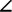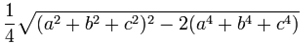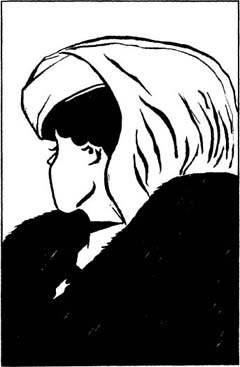# The triangle paradox – solved

When I posted The triangle paradox, I said that I would post a solution in few days. As per the comments on my earlier article, some via Twitter and indeed the context of the article in which this supposed mathematical conundrum was posted, the heart of the matter is an optical illusion.

If we consider just the first part of the paradox:Then the key is in realising that the red and green triangles are not similar (in the geometric sense of the word). In particular the left hand angles are not the same, thus when lined-up they do not form the hypotenuse of the larger, compound triangle that our eyes see. In the example above, the line tracing the red and green triangles dips below what would be the hypotenuse of the big triangle. In the rearranged version, it bulges above. This is where the extra white square comes from.

It is probably easier to see this diagrammatically. The following figure has been distorted to make things easier to understand:EAB = tan-1(2/5) ≈ 21.8°FAC = tan-1(3/8) ≈ 20.6°

So the two triangles are not similar and, as stated above, the two arrangements don’t quite line up to form the big triangle shown in the paradox. There is a “gap” between them formed by the grey parallelogram above, whose size has been exaggerated. This difference gets lost in the thickness of the lines and also our eyes just assume that the two arrangements form the same big triangle.

To work out the area of the parallelogram:

AE = (22 + 52)½ = √29
EI = (32 + 82)½ = √73
AI = (52 + 132)½ = √194

The area of a triangle with sides a, b and c is given by:Sparing you the arithmetic, when you substritute the values for AE, EI and AI in the above equation, the area of ∆ AEI is precisely ½.

∆ AEI and ∆ AFI are clearly identical, so the area of parallelogram AEIF is twice the area of either is

2 x ½ = 1

This is where the “missing” square comes from.

As was pointed out in a comment on the original post, the above should form something of a warning to those who place wholly uncritical faith in data visualisation. Much like statistics, while this is a powerful tool in the hands of the expert, it can mislead if used without due care and attention.

This seems to be turning into Mathematics week at peterjamesthomas.com. The “paradox” shown in the latter part of this article was presented to the author and some of his work colleagues at a recent seminar. It kept company with some well-know trompe l’œil such as:andandHowever the final item presented was rather more worrying as it seemed to be less related to the human eye’s (or perhaps more accurately the human brain’s) ability to discern shape from minimal cues and more to do with mathematical fallacy. The person presenting these images (actually they were slightly different ones, I have simplified the problem) claimed that they themselves had no idea about the solution.

Consider the following two triangles:The upper one has been decomposed into two smaller triangles – one red, one green – a blue rectangle and a series of purple squares.

These shapes have then been rearranged to form the lower triangle. But something is going wrong here. Where has the additional white square come from?

Without even making recourse to Gödel, surely this result stabs at the heart of Mathematics. What is going on?

After a bit of thought and going down at least one blind alley, I managed to work this one out (and thereby save Mathematics single-handedly). I’ll publish the solution in a later article. Until then, any suggestions are welcome.

For those who don’t want to think about this too much, the solution has now been posted here.